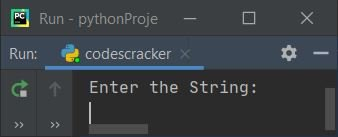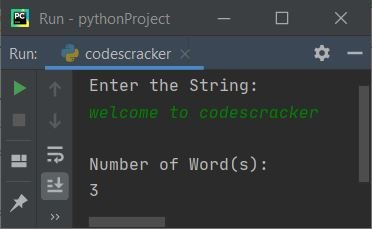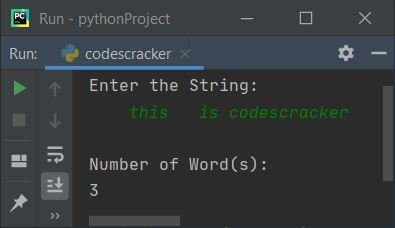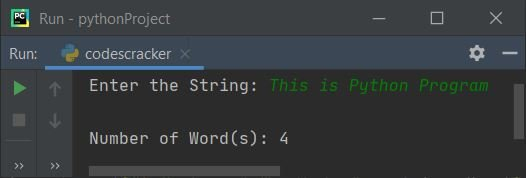# Python Program to Count Words in a String

This article is created to cover some programs in Python, that count and prints total number of words available in a string entered by user at run-time. Here are the list of approaches used to do the job:

• Count Words in String using for Loop
• Using len() and split() Methods
• Using user-defined Function
• Using Class

## Count Words in String using for Loop

To count total number of words present in a given string or sentence in Python, you have to ask from user to enter a string, then count and print total words available in the string like shown in the program given below:

```print("Enter the String: ")
text = input()

chk = 0
countWord = 0
textLen = len(text)

for i in range(textLen):
if text[i]==' ':
if chk!=0:
countWord = countWord+1
chk = 0
else:
chk = chk+1

if chk!=0:
countWord = countWord+1

print("\nNumber of Word(s): ")
print(countWord)```

Here is its sample run:Now supply the input say welcome to codescracker as string and press `ENTER` key to count and print the total number of words available in the given string:Here is another sample run with following user input:

`    this   is codescracker`

That is, 4 spaces then this, 3 spaces then is, and a single space then codescracker:Note - The len() method returns length of string passed as its argument.

In above program, the following code (for loop's code):

`for i in range(textLen):`

is used to execute all statements available in its body, textLen number of times with value of i from 0 to textLen-1. For example, if textLen's value is 5, then this loop gets evaluated five times with value of i from 0 to 4

The dry run of above program with string input welcome to codescracker, as provided in first sample run, goes like:

• Initial values, chk=0, countWord=0, text="welcome to codescracker" (entered by user)
• Now the following statement:
`textLen = len(text)`
initializes the length of string stored in text to textLen. So textLen=23
• Now the execution of for loop begins. Initially i=0, since 0 is less than 23, therefore condition evaluates to be true, so program flow goes inside the loop
• Inside the loop, the condition (of if) text[i]==' ' or text==' ' or 'w'==' ' evaluates to be false, therefore program flow does not goes in its body
• Rather it goes to else's body and chk+1 or 0+1 or 1 gets initialized to chk. So chk=1
• Now the value of i gets incremented. So i=1, since 1 is less than 23, therefore program flow again goes inside the loop
• Again the condition (of if) text[i]==' ' or text==' ' or 'e'==' ' evaluates to be false, therefore chk+1 or 1+1 gets initialized to chk
• Now i=2, since 2 is less than 23, therefore third time also, program flow goes inside the for loop's body
• This process continues, until the condition evaluates to be false, or until the value of i becomes equal to 23 (length of string)
• Inside the loop, when character at any ith index gets equal to a space, then I've checked whether the value of chk is not equal to 0 or not, if condition evaluates to be true, then only the variable countWord gets incremented by 1
• In this way, after exiting from the loop, I've a variable named countWord that holds the total number of words
• After exiting from the loop, another condition applied to check and increment the value of countWord for last word. Because in general, last word in any sentence or string does not contains any space after it.
• Now print the value of countWord as number of words available in the string entered by user.

## Count Words in String using len() and split()

This program also count and prints total words available in a string, but using predefined function named split(). The split() method splits string into words. Let's have a look at the program first:

```print(end="Enter the String: ")
text = input()

wordLen = len(text.split())
print("\nNumber of Word(s): " + str(wordLen))```

Here is its sample run with user input, This is Python Program:From above program, the following code (statement):

`wordLen = len(text.split())`

can also be replaced with the block of code given below, to make it more understandable:

```wordlist = text.split()
wordLen = len(wordlist)```

that is, the first statement creates a list of words using split(). And the second statement initializes the length of list named wordlist using len() method. Length of list means, total number of elements available in the list. Elements of list here are words

## Count Words in a String using Function

This program uses a user-defined function named CountWords(), that receives a string as its argument and returns its length like done in previous program. The only difference is, the main code gets wrapped into a user-defined function.

```def CountWords(s):
wlist = s.split()
wtot = len(wlist)
return wtot

print("Enter the String: ", end="")
text = input()

wordLen = CountWords(text)
print("\nNumber of Word(s):", wordLen)```

Produces same output as of previous program.

## Count Words in a String using Class

This is the last program created using a class named CodesCracker. Class is an object-oriented feature of Python. Function inside a class, is called as its member function.

```class CodesCracker:
def CountWords(self, s):
wrds = s.split()
totwrds = len(wrds)

print("Enter the String: ", end="")
text = input()

ob = CodesCracker()
textlen = ob.CountWords(text)
print("\nNumber of Word(s):", textlen)```

To access member function of a class, an object of that class is required. Therefore an object named ob of class CodesCracker is created, and using dot (.) operator, I've accessed its member function through its object like shown in above program.

#### Same Program in Other Languages

Python Online Test

« Previous Program Next Program »